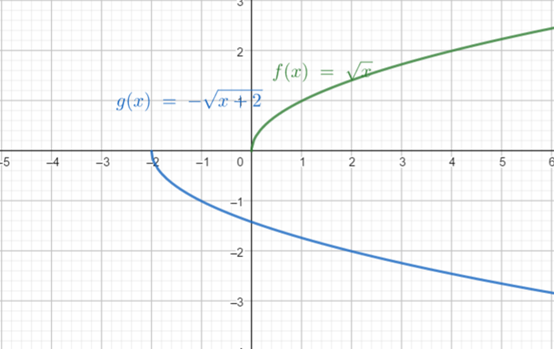# the formula of function g .### Precalculus: Mathematics for Calcu...

6th Edition
Stewart + 5 others
Publisher: Cengage Learning
ISBN: 9780840068071### Precalculus: Mathematics for Calcu...

6th Edition
Stewart + 5 others
Publisher: Cengage Learning
ISBN: 9780840068071

#### Solutions

Chapter 2.5, Problem 59E
To determine

## To find : the formula of function g .

Expert Solution

The function is g=x+2 .

### Explanation of Solution

Given information : the function is f=xForm the above graph it is observed that the graph of the function of g is the graph of f=x shifted 2 unit left and then reflected in the x -axis.

Use the definition of horizontally shifts and reflecting graphs,

Then for the function of gsubstitute x by (x+2) in the function f=x then multiply by -1.

Hence, the function g=x+2 .

### Have a homework question?

Subscribe to bartleby learn! Ask subject matter experts 30 homework questions each month. Plus, you’ll have access to millions of step-by-step textbook answers!